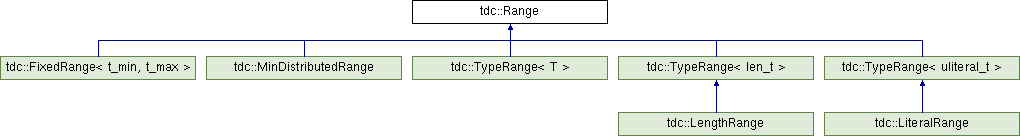tudocomp – The TU Dortmund Compression Framework
tdc::Range Class Reference

Represents a generic range of positive integers. More...

`#include <Range.hpp>`

Inheritance diagram for tdc::Range:## Public Member Functions

constexpr Range (size_t max)
Constructs a range from zero to a maximum value. More...

constexpr Range (size_t min, size_t max)
Constructs a range. More...

size_t min () const
Yields the range's minimum value. More...

size_t max () const
Yields the range's maximum value. More...

size_t delta () const
Yields the difference between the range's minimum and maximum values. More...

## Detailed Description

Represents a generic range of positive integers.

Ranges have a minimum and a maximum value. They are used as hints for a Encoder or Decoder for it to determine how to encode/decode certain values.

Correspondingly implemented coders can use this information to encode more efficiently, therefore it is advised to feed it ranges as precise as possible for every value to encode.

Definition at line 16 of file Range.hpp.

## ◆ Range() [1/2]

 constexpr tdc::Range::Range ( size_t max )
inline

Constructs a range from zero to a maximum value.

Parameters
 max the range's maximum value

Definition at line 24 of file Range.hpp.

## ◆ Range() [2/2]

 constexpr tdc::Range::Range ( size_t min, size_t max )
inline

Constructs a range.

Parameters
 min the range's minimum value max the range's maximum value

Definition at line 31 of file Range.hpp.

## ◆ delta()

 size_t tdc::Range::delta ( ) const
inline

Yields the difference between the range's minimum and maximum values.

Returns
the difference between the range's minimum and maximum values

Definition at line 47 of file Range.hpp.

## ◆ max()

 size_t tdc::Range::max ( ) const
inline

Yields the range's maximum value.

Returns
the range's maximum value

Definition at line 41 of file Range.hpp.

## ◆ min()

 size_t tdc::Range::min ( ) const
inline

Yields the range's minimum value.

Returns
the range's minimum value

Definition at line 37 of file Range.hpp.

The documentation for this class was generated from the following file: